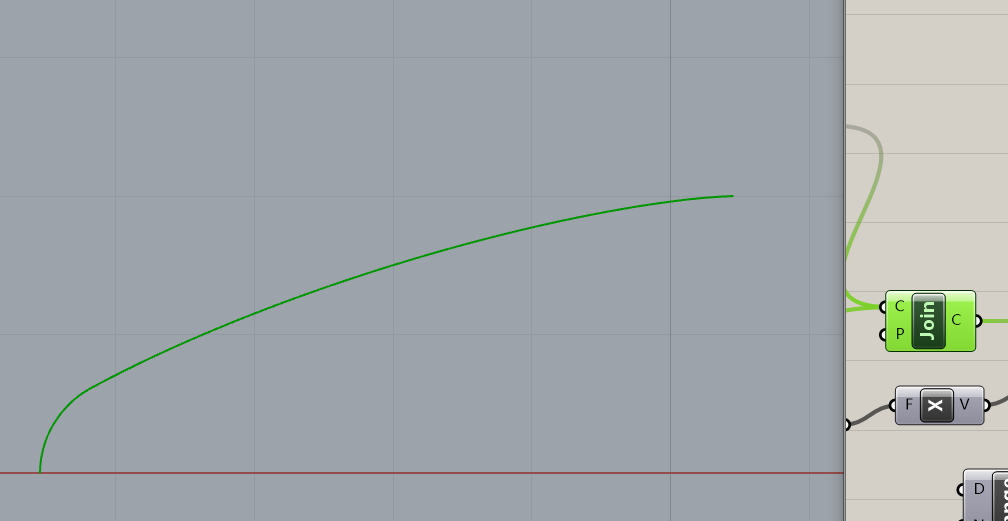## 思路

$\theta=\arccos(1-\frac{2x}{L})\\ r(\theta)=\frac{R}{\sqrt{\pi}}\sqrt{\theta-\frac{\sin 2\theta}{2}}$

• 绘制冯·卡门曲线
• 取断点，求断点处斜率
• 根据斜率计算圆弧大小与位置

• 计算总长
• 根据总长自动修正参数（L）

## 实现

r 输出经过修正的 L 长度，a 则输出圆心位置。